Like   Tweet   Pin# IN THIS ISSUE:

▪ Dayton "Dirt" Blog for June 7th
▪ The Hairy Balls are Back!
▪ The Mother of Thousands are Back!
▪ June Gardening To-Do List
 ▪ Dayton "Dirt" Blog for June 7th
 ▪ The Hairy Balls are Back!
 ▪ The Mother of Thousands are Back!
 ▪ June Gardening To-Do List
 table div table+table+table div table{width:100%;padding:0}table div table+table+table div table img{width:96.23%;padding:0;float:none}table div table+table+table div table td{width:100%;padding:0 1.88% 18px}/* styles */## DAYTON "DIRT" BLOG - June 7, 2019

 /* styles */ No doubt with the 2-4 plus inches of rain this past week, newly planted flowers and gardens will definitely not need watered and hopefully the rainy period will abate with some sunshine and a good weather pattern of one inch of rain per week. The newly planted trees and shrubs here at the nursery are growing nicely especially with the advantage of the higher quality of the rain water as compared to irrigation. Igloo Mum starts also arrived this week and we are potting them up into larger pots for fall sales. Believe it or not, these little plants will be full and lush (and blooming) by mid August!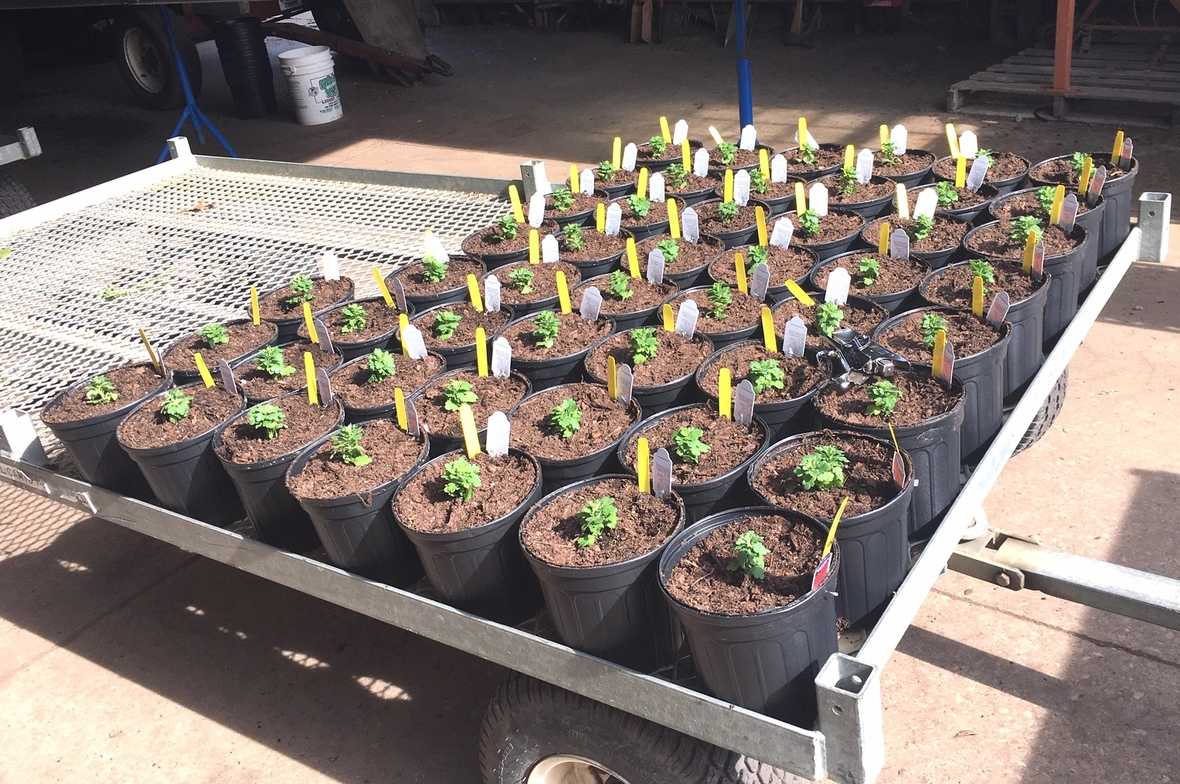/* styles */ In the greenhouse, potted flowers of every sort still abound as well as hanging baskets as the benefit from the higher rate of fertilizer that is needed for the higher growth rate during the long sunny (sometime sunny) days of June is evident with floriferous blooms and rapid growth.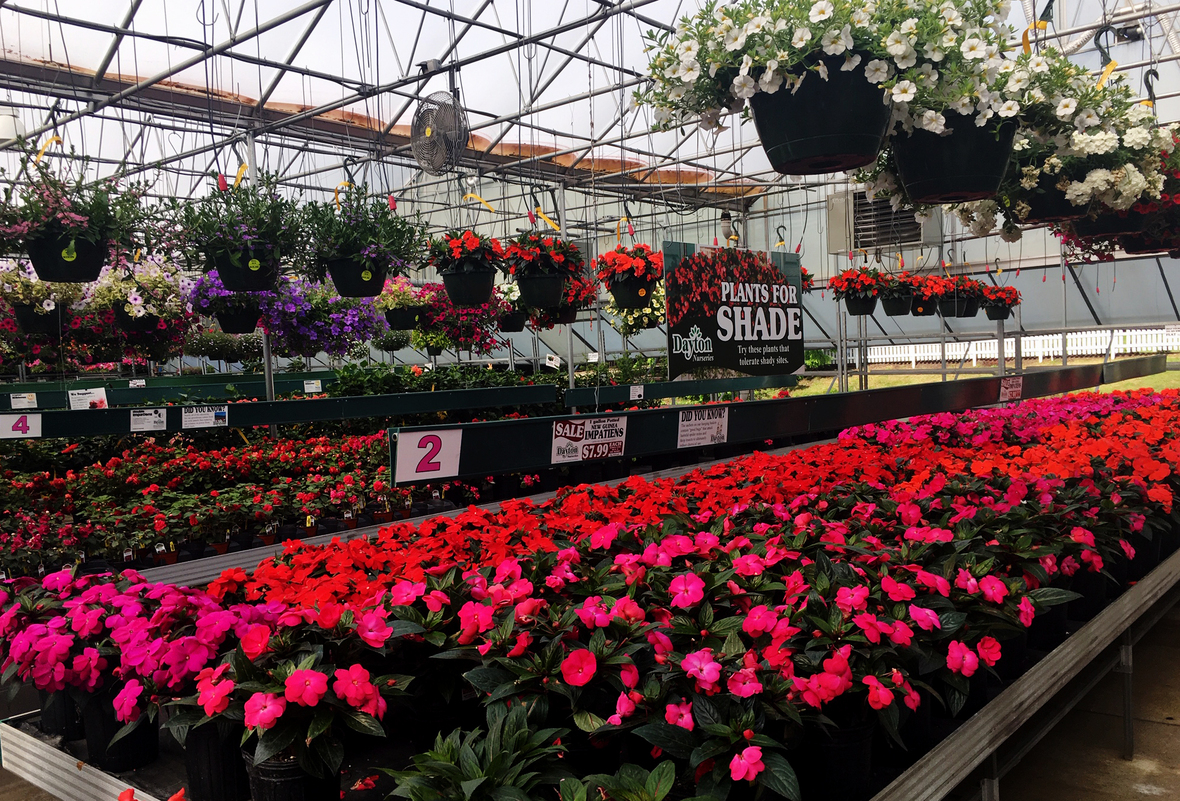/* styles */
 /* styles */ Now is the time to plant another patch of beans, cucumbers and whatever vegetable you would like to achieve successive planting for a continued harvest through summer and late fall. The lawns seem to be thriving as water and cool temperatures mimic spring and the high growth rate. No doubt, plants will grow, but the weeds even more. Happy weeding! Tom
 table div table+table+table+table+table+table+table+table+table+table+table div table{width:100%;padding:0}table div table+table+table+table+table+table+table+table+table+table+table div table img{width:96.23%;padding:0;float:none}table div table+table+table+table+table+table+table+table+table+table+table div table td{width:100%;padding:0 1.88% 18px}/* styles */## THEY'RE BACK!

 /* styles */ Many of you have been asking.... and yes, the hairy balls balloon milkweeds are finally ready!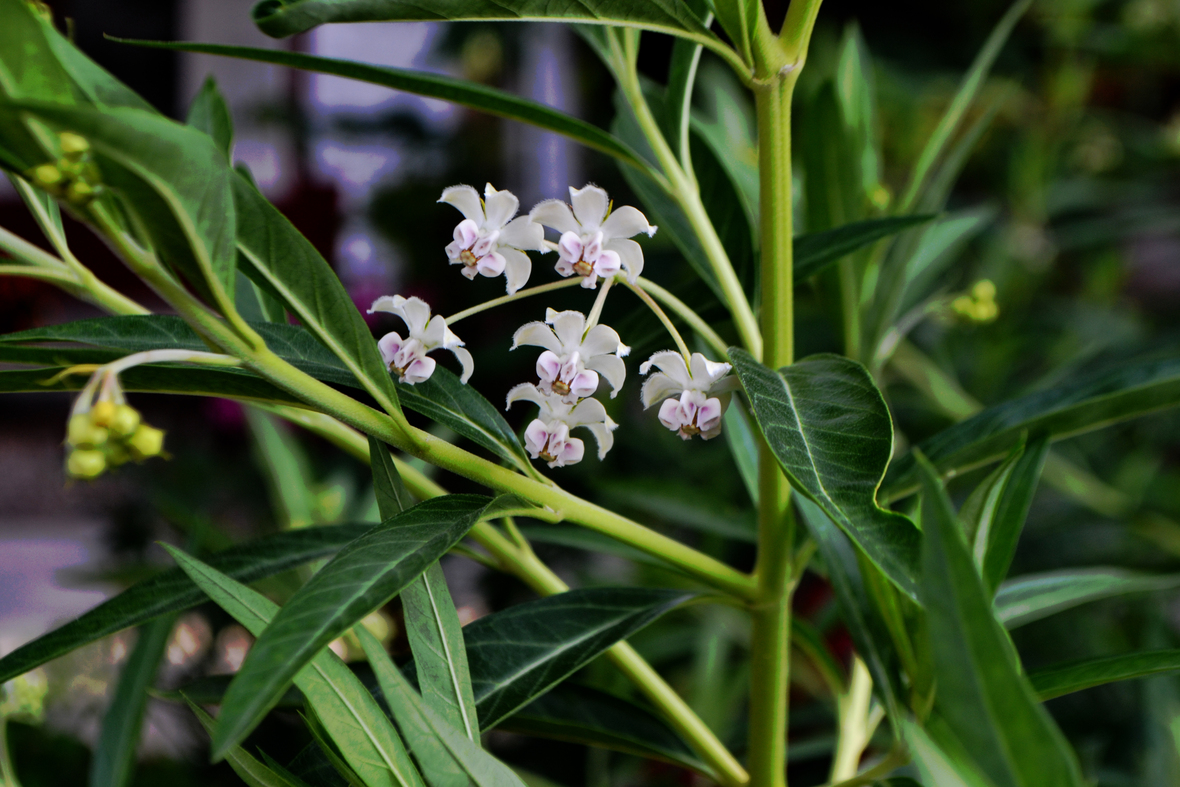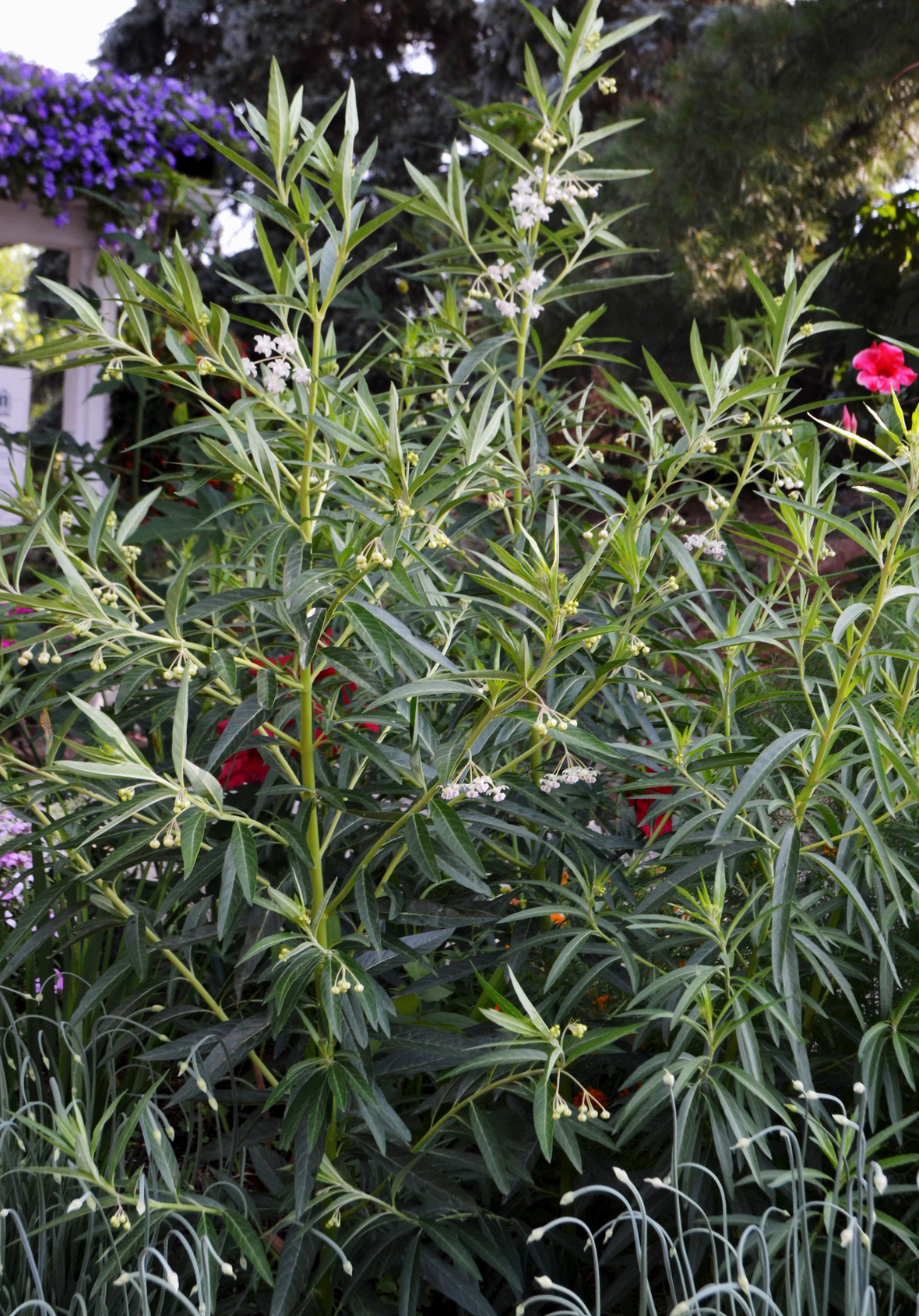/* styles */ The Hairy Balls plant, Gomphocarpus physocarpus, is also known as Goose plant, balloon milkweed, balloon plant & even more names that may sound quite inappropriate so I will leave those out of this email! This plant is a MUST for those wanting to attract butterflies and help with the population of the Monarch butterfly. In our experience, this plant is by far their favorite to munch on and lay eggs. This is also the ideal plant to cut branches and use for monarch caterpillar hatching indoors. Be sure to plant more than one as the caterpillars could munch down an entire plant in just one afternoon! This ANNUAL milkweed can grow up to 4-6' tall with blooms mid to late summer followed by showy, round seed pods. This plant is a real conversation piece! They are available in a 4" pot for \$4.99 each. Check below to save \$1.00 off each when you buy 3 or more!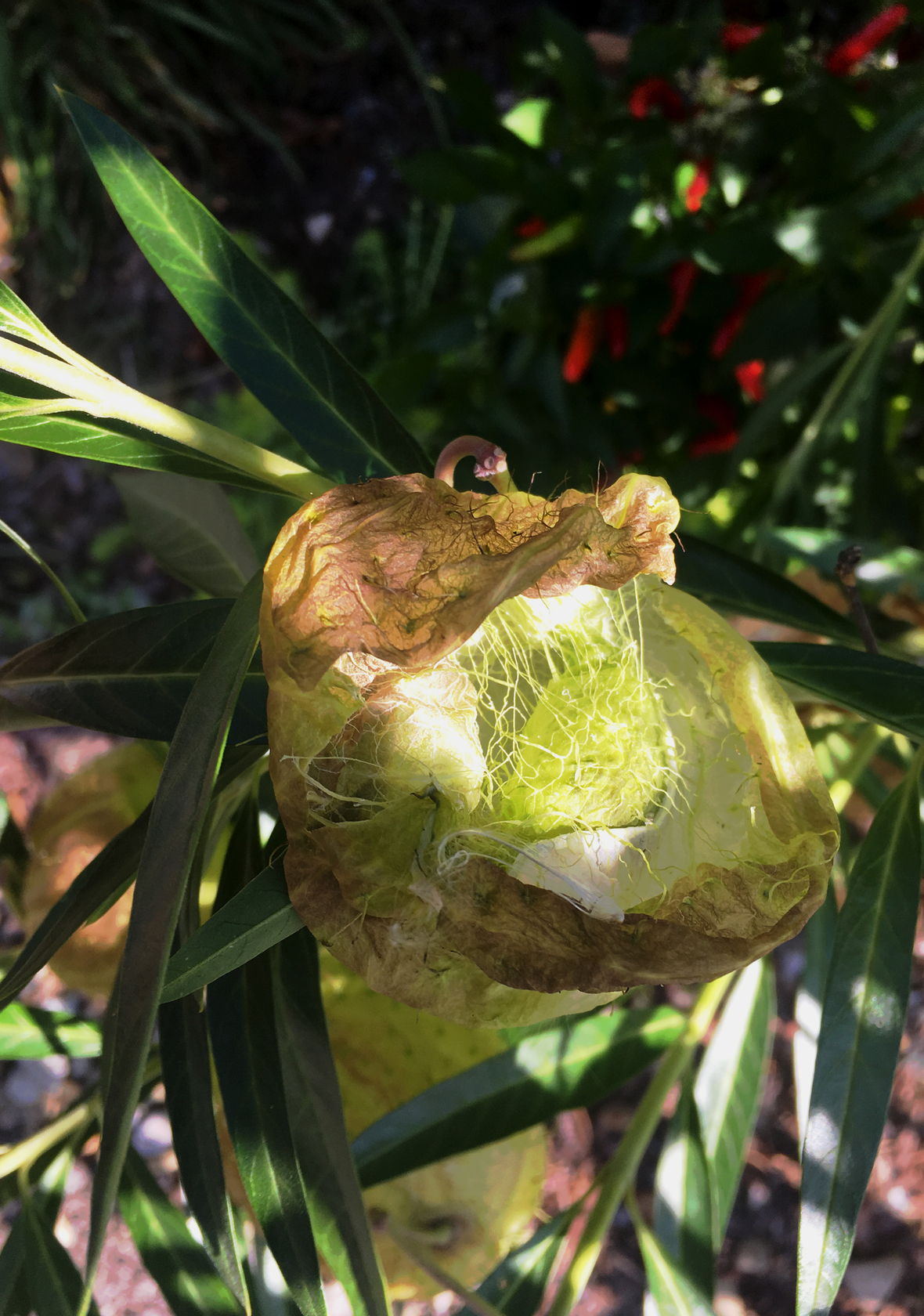Not Ready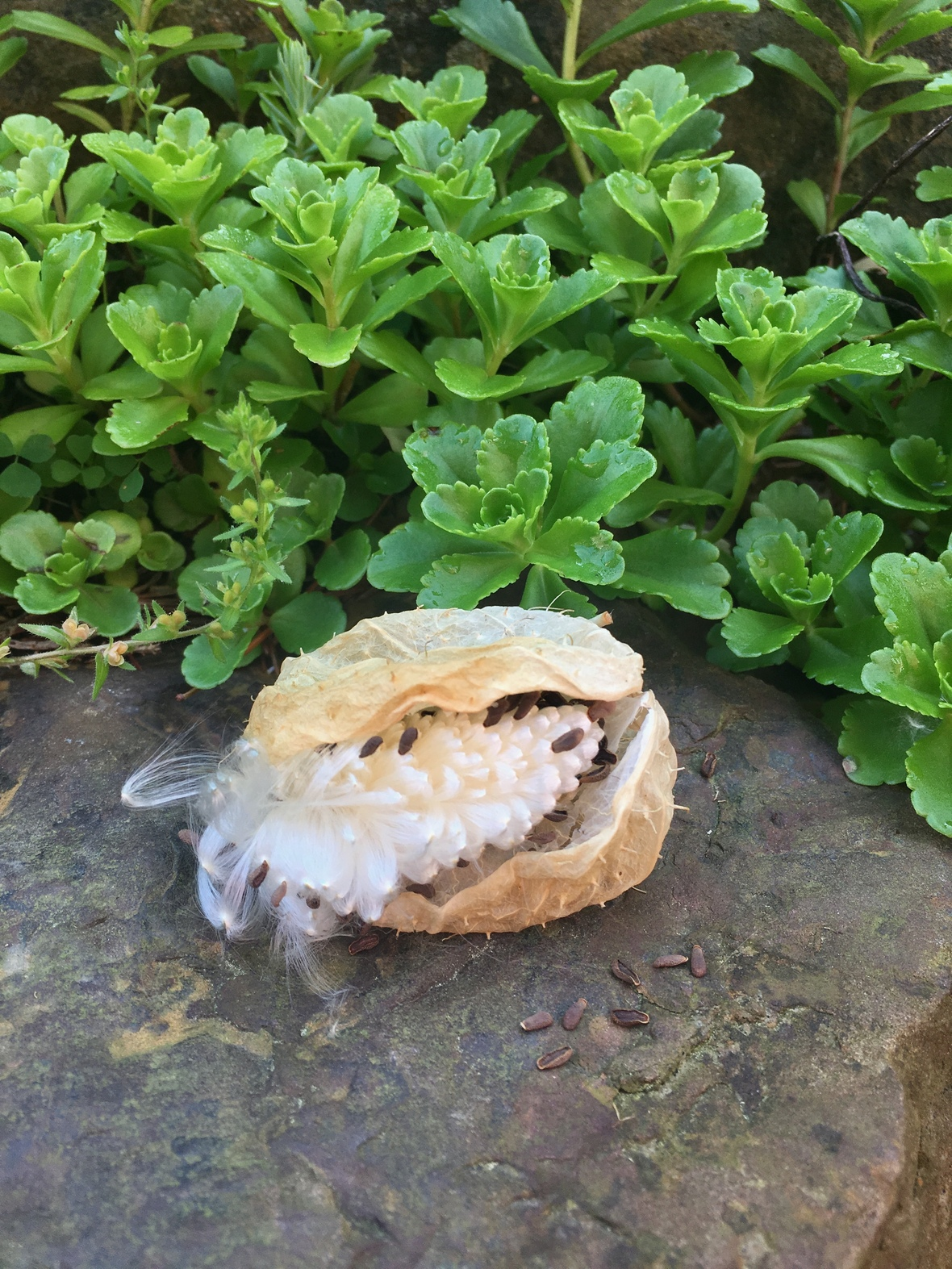Ready
 /* styles */ Want to share with friends next year? You can retrieve the seeds when ready and store them until spring to start about 2 months before the final frost date of the season. As shown above, do not force open the seed pods (left pic). They will start to open when they look drier and blackened (right pic). At this time the seeds are ready to harvest.table div table+table+table+table+table+table+table+table+table+table+table+table+table+table+table+table+table+table+table div table{width:100%;padding:0}table div table+table+table+table+table+table+table+table+table+table+table+table+table+table+table+table+table+table+table div table img{width:96.23%;padding:0;float:none}table div table+table+table+table+table+table+table+table+table+table+table+table+table+table+table+table+table+table+table div table td{width:100%;padding:0 1.88% 18px}/* styles */## AND, THESE ARE BACK TOO!

 /* styles */ The Mother of Thousands plant (Bryophyllum daigremontianum) was all the rage this year and they sold like hot cakes! Well, we grew some more and they are back in stock. They are in 4" pots for \$7.99 each. Not only do these look really neat but this yet another plant you can easily share with friends! Those little "babies" that form atop of the larger leaves can be plucked off and planted individually into a potting mix for cactus and they will root in and grow quite quickly. What a fun plant to have!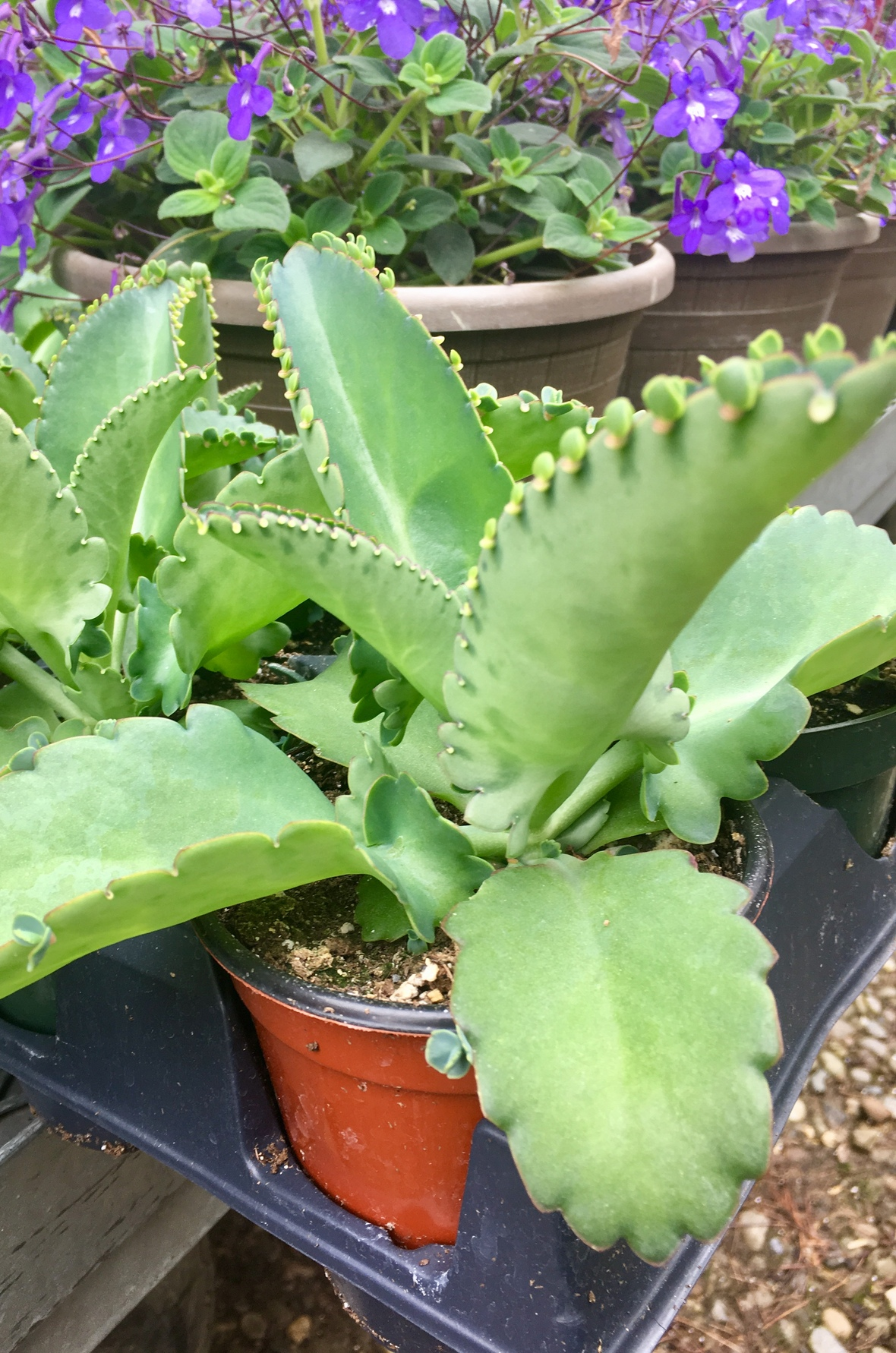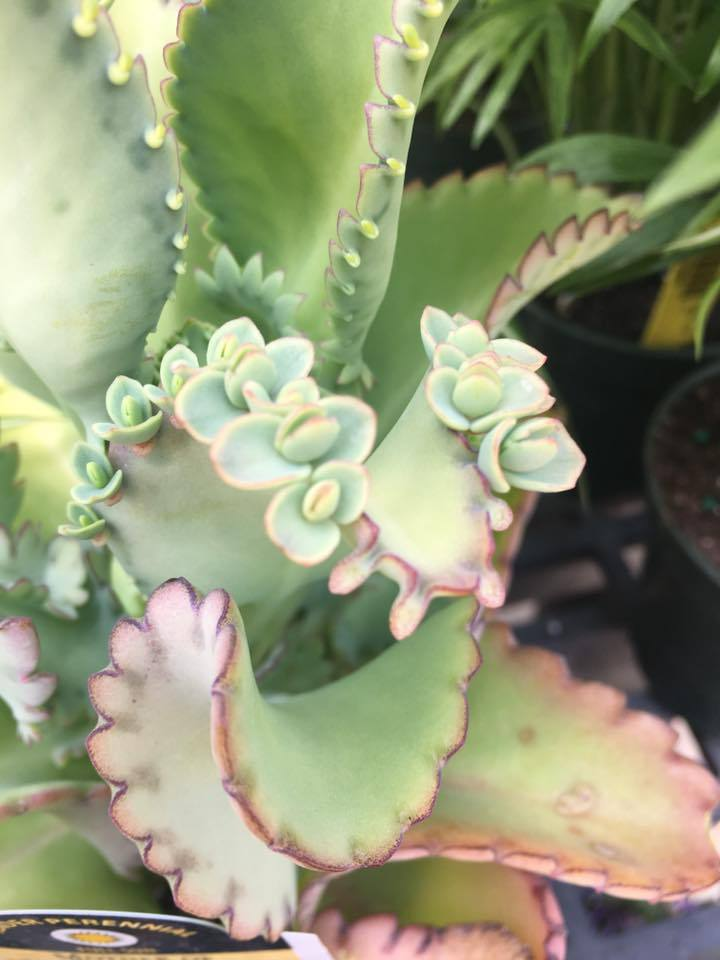table div table+table+table+table+table+table+table+table+table+table+table+table+table+table+table+table+table+table+table+table+table+table+table div table{width:100%;padding:0}table div table+table+table+table+table+table+table+table+table+table+table+table+table+table+table+table+table+table+table+table+table+table+table div table img{width:96.23%;padding:0;float:none}table div table+table+table+table+table+table+table+table+table+table+table+table+table+table+table+table+table+table+table+table+table+table+table div table td{width:100%;padding:0 1.88% 18px}/* styles */## SALES & SPECIALS

 /* styles */ Below are the newest sales and specials to hit Dayton's but there are many more to view, so please click HERE for all current sales & specials!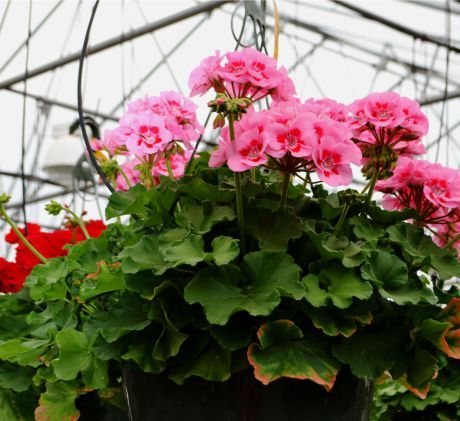Includes all sizes; includes veggie and flower flats

SALE – Buy One, Get One FREE!

 /* styles */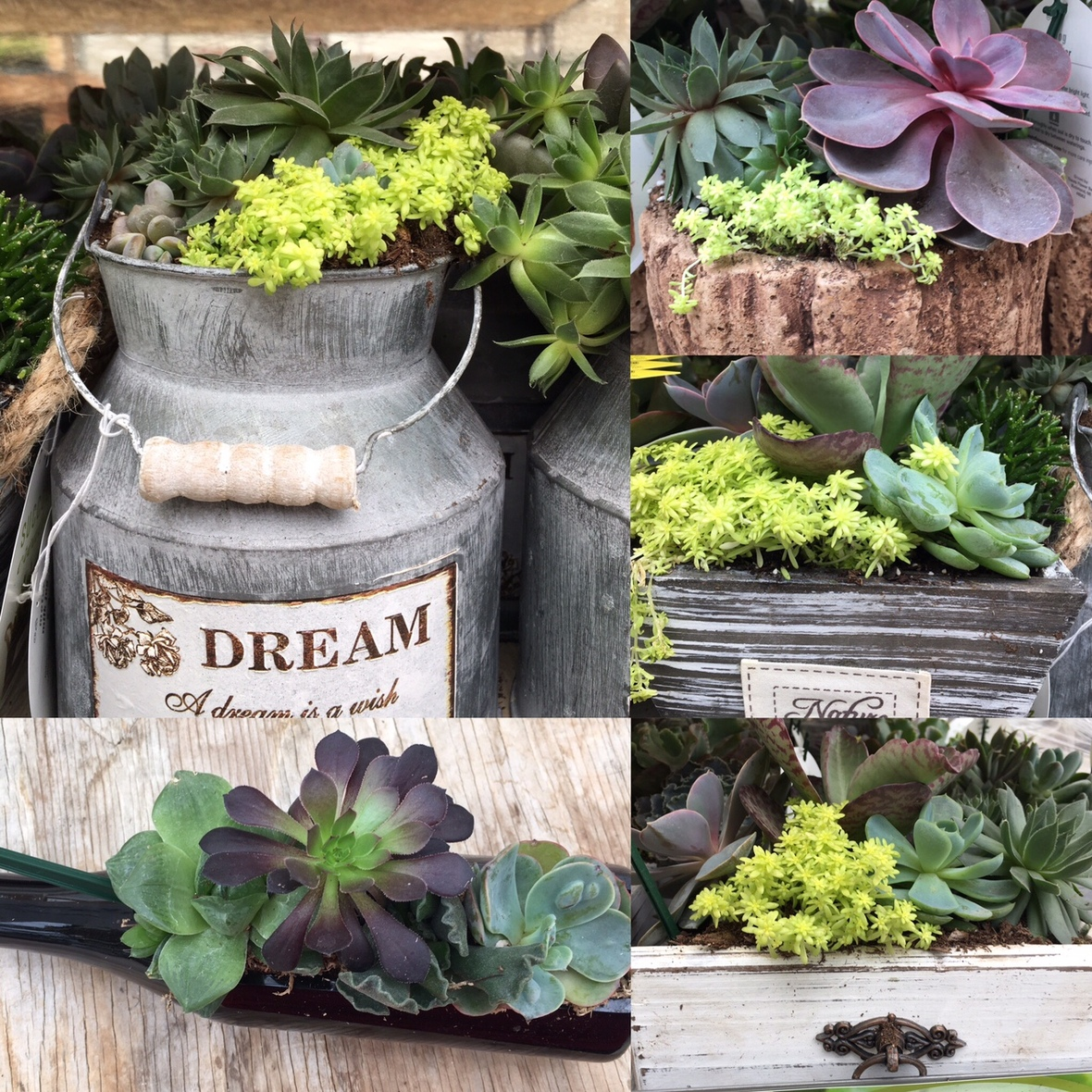Succulents
All sizes & types
Also includes small potted terrarium plants

SALE – 25% OFF

 /* styles */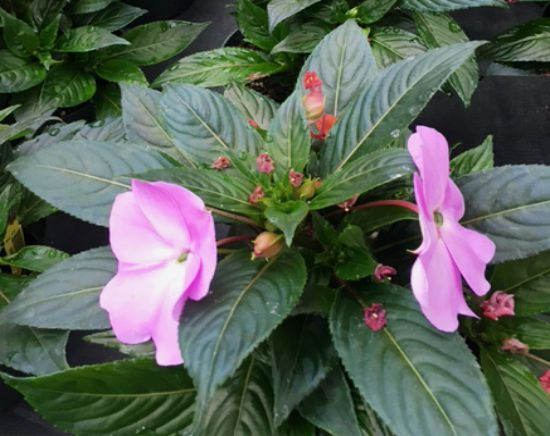New Guinea Impatiens
_large 1 gallon pot size – Reg. \$9.99 each.

SALE – Just \$7.99 each when you buy 3 or more

 /* styles */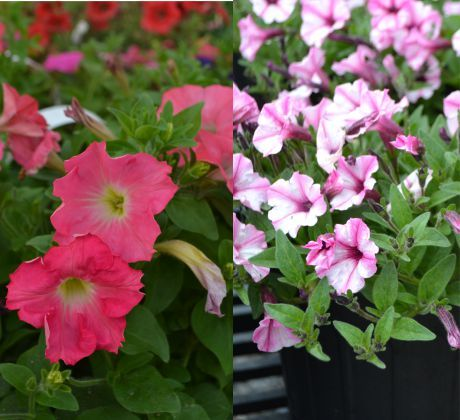8” Potted Annuals
8” pot; reg. \$7.99 each; includes Calibrachoa #26870, Petunia #27590, Verbena #26869

SALE – BUY 5 or more for \$6.99 each

 /* styles */
 table div table+table+table+table+table+table+table+table+table+table+table+table+table+table+table+table+table+table+table+table+table+table+table+table+table+table+table+table+table+table+table+table+table+table+table div table{width:100%;padding:0}table div table+table+table+table+table+table+table+table+table+table+table+table+table+table+table+table+table+table+table+table+table+table+table+table+table+table+table+table+table+table+table+table+table+table+table div table img{width:96.23%;padding:0;float:none}table div table+table+table+table+table+table+table+table+table+table+table+table+table+table+table+table+table+table+table+table+table+table+table+table+table+table+table+table+table+table+table+table+table+table+table div table td{width:100%;padding:0 1.88% 18px}/* styles */table div table+table+table+table+table+table+table+table+table+table+table+table+table+table+table+table+table+table+table+table+table+table+table+table+table+table+table+table+table+table+table+table+table+table+table+table+table+table+table div table{width:100%;padding:0}table div table+table+table+table+table+table+table+table+table+table+table+table+table+table+table+table+table+table+table+table+table+table+table+table+table+table+table+table+table+table+table+table+table+table+table+table+table+table+table div table img{width:96.23%;padding:0;float:none}table div table+table+table+table+table+table+table+table+table+table+table+table+table+table+table+table+table+table+table+table+table+table+table+table+table+table+table+table+table+table+table+table+table+table+table+table+table+table+table div table td{width:100%;padding:0 1.88% 18px}/* styles */table div table+table+table+table+table+table+table+table+table+table+table+table+table+table+table+table+table+table+table+table+table+table+table+table+table+table+table+table+table+table+table+table+table+table+table+table+table+table+table+table+table+table div table{width:100%;padding:0}table div table+table+table+table+table+table+table+table+table+table+table+table+table+table+table+table+table+table+table+table+table+table+table+table+table+table+table+table+table+table+table+table+table+table+table+table+table+table+table+table+table+table div table img{width:96.23%;padding:0;float:none}table div table+table+table+table+table+table+table+table+table+table+table+table+table+table+table+table+table+table+table+table+table+table+table+table+table+table+table+table+table+table+table+table+table+table+table+table+table+table+table+table+table+table div table td{width:100%;padding:0 1.88% 18px}/* styles */